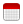Your browser is not optimized for viewing this website.

# College and Career Readiness Standards (CCRS) Math

## Video - CCRS Math (101) A Introduction & Key Instructional Shifts

Free

with ATDN StaffJun 30, 2021 at 8:30 am

CCRS Math 101(A) Introduction and Key Instructional Shifts (Video session) Part 1 of 4 videos.

The College and Career Readiness Standards (CCRS) for Adult Education are a set of academic standards that, when met by students, prepare adult learners for success in college, technical training program(s), and work.  In this session, participants will become familiar with the standards and how to navigate them.  The video will investigate the Key Instructional Shifts mathematics instruction must make in order for students to succeed.  The importance of taking the time to delve deeply into the concepts at the heart of mathematics will be illustrated.  By making connections between mathematical concepts, activities will encourage ways to see mathematics as more than discrete sets of rules to be memorized.

Purpose:

Introduce the Key Instructional Shifts of the College and Career Readiness Standards for Adult Education

Develop a working knowledge of the Key Instructional Shifts

Explore how the Shifts influence the structure of the CCR content standards and the order in which standards should be taught

Consider how Shifting practice unfolds in the classroom.

Will run

## Video - CCRS Math (102) A Standards for Mathematical Practice

Free

with ATDN StaffJun 30, 2021 at 8:30 am

CCRS Math 102(A) Standards for Mathematical Practice (video session) Part 3 of 4 videos. Building on work done in the CCRS Math 101 video, participants will experience problems worth puzzling through and analyze the habits of mind required to solve those problems.  We will investigate Practices through activities that illustrate the ways students are to engage with the subject matter of the content standards.  The skills of complex problem solving, reasoning and proof, precise communication, and making connections will be practiced and discussed.  Activities will encourage a deeper understanding of how to implement CCRS in instruction.

Purpose:

Develop a working understanding of the Standards for Mathematical Practice

Explore how the Standards for Mathematical Practice influence the CCR content standards and how those standards should be taught

Consider ways and resources to elicit Standards for Mathematical Practice from students

Participants must have completed Math 101A & Math 101B  before attending Math 102(A).

Will run

## Video - CCRS Math (101) B Key Instructional Shifts

Free

with ATDN StaffJun 30, 2021 at 1 pm

CCRS Math 101(B) Key Instructional Shifts (video) Part 2 of 4 videos. Building on the work of 101(A), participants will become familiar with the standards and how to navigate them.  We will continue to investigate the Key Instructional Shifts mathematics instruction must make in order for students to succeed.  The importance of taking the time to delve deeply into the concepts at the heart of mathematics will be illustrated.  By making connections between mathematical concepts, activities will encourage ways to see mathematics as more than discrete sets of rules to be memorized.  The content standards calling for a balance of conceptual understanding, fluency with procedures, and application to real-world and mathematical contexts will be explored.  Participants will leave with a deeper understanding of how to use standards when planning math instruction.

Purpose:

Develop a working knowledge of the Key Instructional Shifts

Consider how Shifting practice unfolds in the classroom

Examine the Key Instructional Shifts when designing instruction for adult learners

Participants must have completed Math 101(A) before attending Math 101(B).

Will run

## Video - CCRS Math (102) B Standards for Mathematical Practice

Free

with ATDN StaffJun 30, 2021 at 1 pm

CCRS Math 102(B) Standards for Mathematical Practice (vido session) Part 4 of 4 videos. Building on work of the CCRS Math 102(A) video, participants will experience problems worth puzzling through and analyze the habits of mind required to solve those problems.  The video will investigate Practices through activities that illustrate the ways students are to engage with the subject matter of the content standards.  The skills of complex problem solving, modeling, precise communication, and making connections will be practiced and discussed.  Activities will encourage a deeper understanding of how to implement CCRS in instruction.  Participants will create a standards-based lesson plan or draft unit outline that integrates both the Key Instructional Shifts and the Standards for Mathematical Practice with priority content standards.

Assignment: Unit Plan Purpose:

Develop a working understanding of the Standards for Mathematical Practice

Consider ways and resources to elicit Standards for Mathematical Practice from students

Examine the Standards for Mathematical Practice when designing instruction

Participants must have completed Math 101(A) & 101(B) and Math 102(A) before attending Math 102(B).

Will run